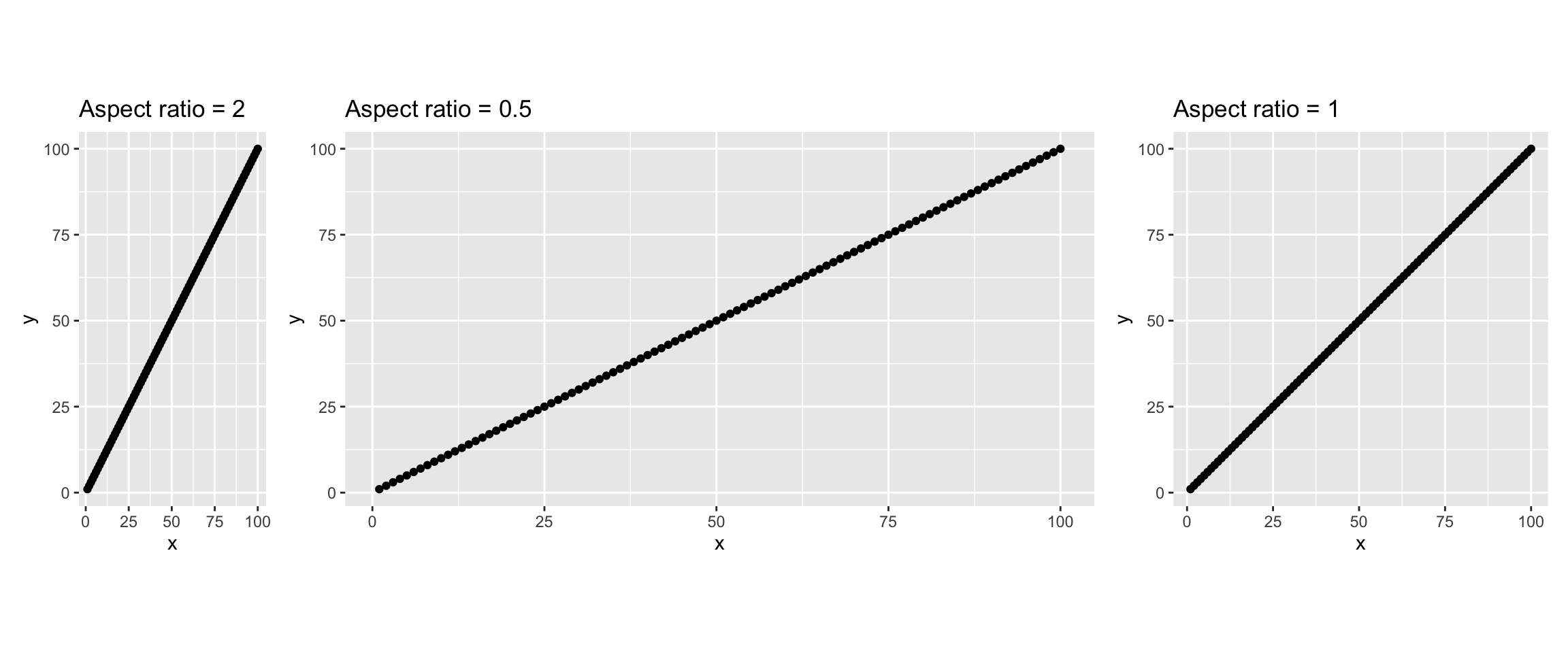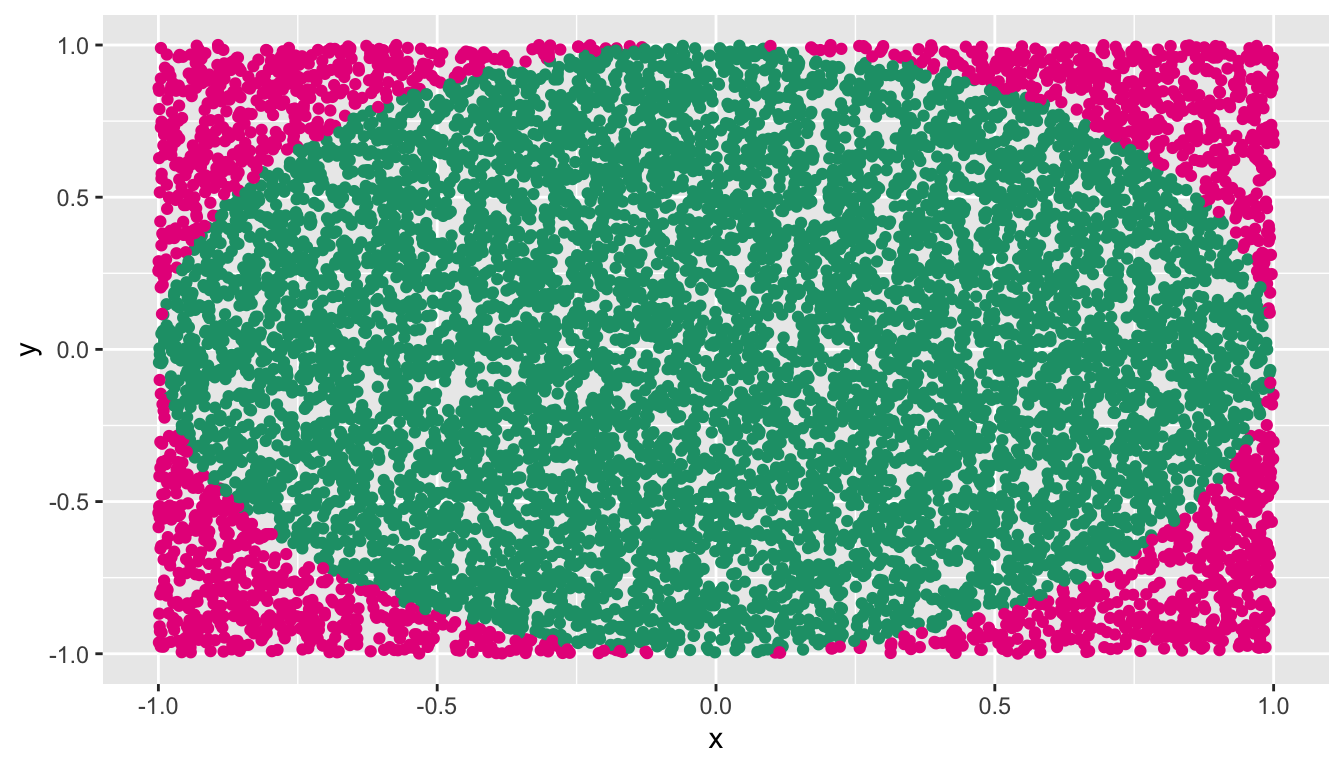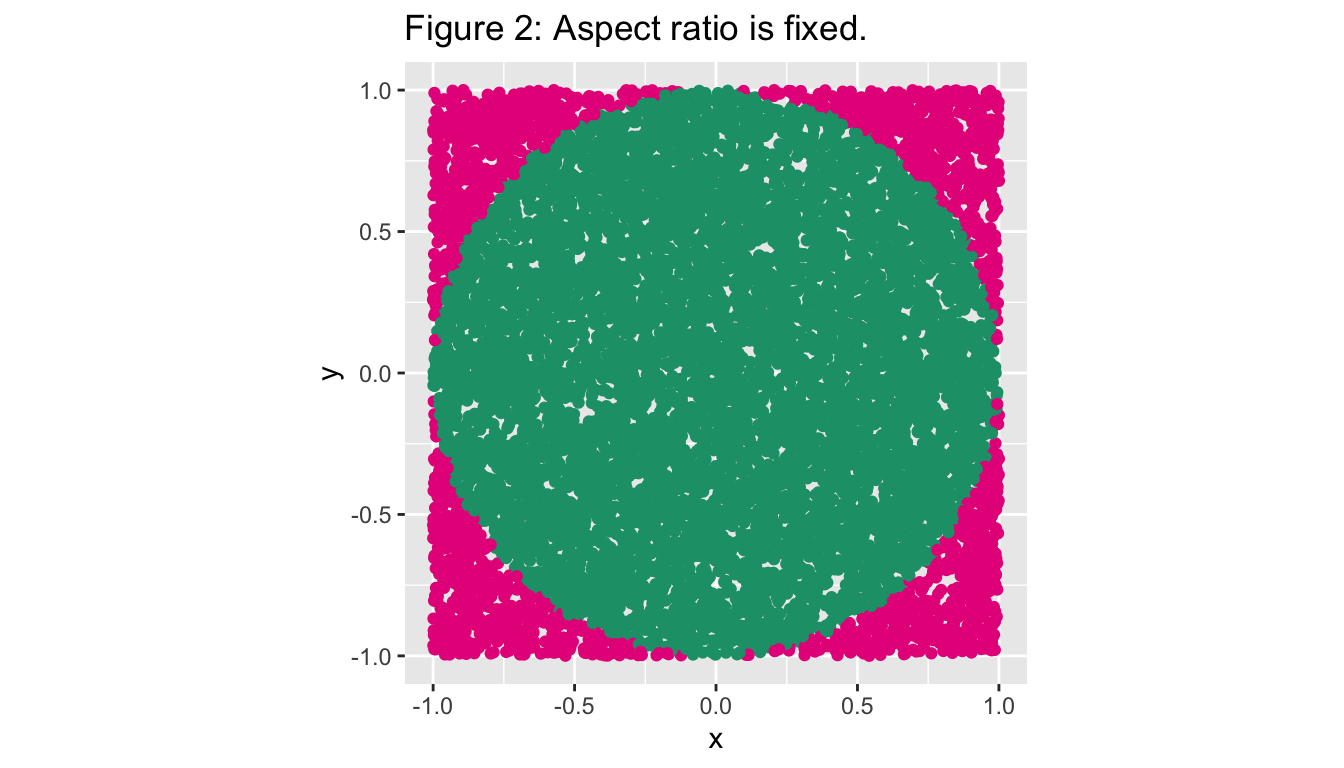# Ratio matters: change the way you see things!

### Aspect ratio

The aspect ratio of a plot is the ratio of its height-to-width . The aspect ratio we choose for our graph plays a significant role. The graphs below plot exactly the same data points but they have different aspect ratios.## The aspect-ratio of a plot can make an influence on the reader’s mind.

Here is an example. I generate a set of random points inside a unit circle. The equation of the unit circle center at (0, 0) is: $$x^2+y^2=1$$.

set.seed(2020)
x <- runif(10000, -1, 1)
y <- runif(10000, -1, 1)
fx <- x^2 + y^2
coly <-  ifelse(fx <= 1, 1, 0)
coly <- as.factor(coly)
circle.points <- data.frame(x=x, y=y, coly=coly)
head(circle.points)
            x          y coly
1  0.29380568 -0.7572887    1
2 -0.21154848  0.2479973    1
3  0.23700363  0.4911537    1
4 -0.04621773  0.3838934    1
5 -0.72780563  0.8263328    0
6 -0.86523123 -0.3060675    1

### Without fixing aspect ratio

ggplot(circle.points, aes(x=x, y=y, col=coly)) +
geom_point() +
scale_colour_manual(values = c("#e7298a", "#1b9e77")) +
theme(legend.position = "none")According to the plot above it seems that the region is bounded by a ellipse. However, our points are not generated from an elliptical region.

### Aspect ratio is fixed

ggplot(circle.points, aes(x=x, y=y, col=coly)) + geom_point() +
scale_colour_manual(values = c("#e7298a", "#1b9e77")) +
coord_equal() +
ggtitle("Figure 2: Aspect ratio is fixed.") +
theme(legend.position = "none")When you create the plot by preserving the aspect ratio the region is bounded by a circle.